J. Sens. Sens. Syst., 9, 1–6, 2020
https://doi.org/10.5194/jsss-9-1-2020

Special issue: Sensors and Measurement Systems 2019

J. Sens. Sens. Syst., 9, 1–6, 2020
https://doi.org/10.5194/jsss-9-1-2020

Review paper 06 Jan 2020

Review paper | 06 Jan 2020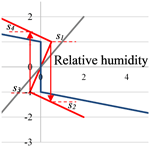# Modelling and model verification of an autonomous threshold sensor for humidity measurements

Modelling and model verification of an autonomous threshold sensor for humidity measurements
Nikolai Gulnizkij and Gerald Gerlach Nikolai Gulnizkij and Gerald Gerlach
• Solid-State Electronics Laboratory, TU Dresden, Dresden, 01062, Germany

Correspondence: Nikolai Gulnizkij (nikolai.gulnizkij@tu-dresden.de)

Abstract

Autonomous sensors that receive their energy from an energy harvester or directly from the environment have the potential to save energy for applications in numerous sectors. For humidity sensing, the swelling behaviour of a water vapour-sensitive hydrogel is used. To trigger an electrical contact the mechanical energy is taken from the hydrogel swelling by the bimorph effect. As long as a defined threshold is not reached, the electrical microcontact remains open. By passing the threshold value, a switching will be triggered causing the closure of the contact. This sensor principle does not need any electrical power supply because the switching power is provided directly by the surrounding humidity as quantity to be measured. For the description of the deflection versus the hydrogel pattern of such a sensor, a model was developed by means of the beam theory. Goal of the model was to derive design guidelines for the dimensioning of the sensors bending plate and the patterned hydrogel layer. Experiments then should show the applicability of the model approaches. The deflection of the bending plate depends on the ratio between the lengths of the uncoated and the coated part of the bending plate where the maximum occurs at a ratio of ca. 0.5. The swelling behaviour of the hydrogel shows a high sensitivity with regard to slight changes in relative humidity. This can be used for humidity threshold sensors that open and close microcontacts with respect to very small changes in relative humidity. To avoid voltage peaks and arcs during the switching process that could arise and destroy the microcontact, a bistable characteristic with hysteresis is needed. Experiments demonstrate the feasibility of this concept and are in good agreement with the modelling results.

Share
1 Motivation

The recent progress in industrial production, building automation, automobile technology and many other fields leads to an increasing demand on sensors and sensor systems that combine data acquisition and signal processing. Such sensor systems have achieved above-average growth of more than 6 % per year (AMA, 2018) in the sensor and measurement technology industry in recent decades. Temperature and humidity are the most important measured quantities. However, in process control 70 % of all sensors are used as threshold switches and in building monitoring more than 90 %. In Bellmann et al. (2015), a novel approach for a non-powered humidity threshold switch based on the BIZEPS (binary zero-power sensor) principle was proposed (see also Frank et al., 2012; Rangelow et al., 2009; Rittersma, 2002). With this principle, the energy is taken directly from the measured variable. In this work, a humidity threshold sensor switch is considered, where the moisture-dependent swelling of a hydrogel, which is part of a bimorph bending plate, is utilized. The switching hysteresis required to achieve an abrupt closing and opening of the contacts can be realized by applying a pre-deflection as well as an axial compression force. To prove this concept, in this work here a simple macro device is considered where both the pre-stress and the pre-deflection are applied by a screw acting axially on an elastically suspended bending beam coated with a humidity-sensitive hydrogel layer.

2 Setup and working principle

Figure 1 shows the general setup and working principle of MEMS-based moisture threshold switches (Huang et al., 2015; Dai et al., 2013). The silicon-based bending plate with a thickness of ca. $\mathrm{2}×{\mathrm{10}}^{-\mathrm{5}}$ m is located in the middle of a silicon die of ca. $\mathrm{5}×{\mathrm{10}}^{-\mathrm{4}}$ m thickness. In the middle, the thin plate comprises a thicker part – the so-called boss structure – that carries one part of the contact pair to be closed or opened. It ensures that the microcontact remains level and flat even if the bending plate is deflected. The humidity-dependent swelling of the hydrogel-coated silicon plate deflects the rigid centre of the bending plate. The water vapour uptake and, hence, the swelling state depend on the humidity of the surrounding air. When swelling, the hydrogel performs mechanical work and, thus, deflects the bending plate mechanically. That means that the energy is supplied by the environment and provides the opportunity to operate the sensor autonomously without any electrical energy supply. Because the swelling and de-swelling are reversible processes, the device can be used both for switching on and off the contacts, respectively.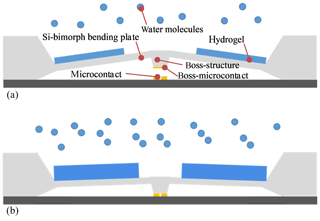Figure 1Humidity threshold sensor switch, consisting of a bimorph bending plate with boss structure, a patterned hydrogel layer and microcontacts, (a) open, (b) closed.

3 Model without axial forces

To calculate the deflection as a function of the hydrogel pattern, a simple mechanical model is used based on the beam theory for monomorph and bimorph beams (Marschner et al., 2014). Figure 2 shows the setup of the sensor and the corresponding mechanical model. The setup consists of the hydrogel-coated part of the bending beam with the torsional compliance nB and the uncoated part of the bending beam with the torsional compliance nP:

$\begin{array}{}\text{(1)}& {n}_{\mathrm{P}}=\frac{\mathrm{12}{l}_{P}}{{E}_{\mathrm{1}}{h}_{\mathrm{1}}^{\mathrm{3}}b},\text{(2)}& {n}_{\mathrm{B}}=\frac{\mathrm{1}}{b}\frac{\mathrm{12}\left(\mathrm{1}+\frac{{E}_{\mathrm{2}}{h}_{\mathrm{2}}}{{E}_{\mathrm{1}}{h}_{\mathrm{1}}}\right){l}_{B}}{{E}_{\mathrm{1}}{h}_{\mathrm{1}}^{\mathrm{3}}\left(\mathrm{1}+\frac{{E}_{\mathrm{2}}^{\mathrm{2}}{h}_{\mathrm{2}}}{{E}_{\mathrm{1}}^{\mathrm{2}}{h}_{\mathrm{1}}}+\frac{{E}_{\mathrm{2}}}{{E}_{\mathrm{1}}}\left(\mathrm{4}\frac{{h}_{\mathrm{2}}}{{h}_{\mathrm{1}}}+\mathrm{6}\frac{{h}_{\mathrm{2}}^{\mathrm{2}}}{{h}_{\mathrm{1}}^{\mathrm{2}}}+\mathrm{4}\frac{{h}_{\mathrm{2}}^{\mathrm{3}}}{{h}_{\mathrm{1}}^{\mathrm{3}}}\right)\right)}.\end{array}$

Hydrogels are much softer than silicon, steel or other spring materials, so that E2E1 applies. Considering that the hydrogel coating and the bending beam have the same thickness h1=h2, Eq. (2) yields a good approximation:

$\begin{array}{}\text{(3)}& {n}_{\mathrm{B}}=\frac{\mathrm{12}{l}_{B}}{{E}_{\mathrm{1}}{h}_{\mathrm{1}}^{\mathrm{3}}b}.\end{array}$

The hydrogel-coated part of the setup acts as a torsional moment source MRH when the relative humidity causes a swelling strain εRH:

$\begin{array}{}\text{(4)}& {M}_{\mathrm{RH}}=\frac{b}{\mathrm{2}}\frac{{E}_{\mathrm{1}}{E}_{\mathrm{2}}{h}_{\mathrm{1}}{h}_{\mathrm{2}}\left({h}_{\mathrm{1}}+{h}_{\mathrm{2}}\right)}{{E}_{\mathrm{1}}{h}_{\mathrm{1}}+{E}_{\mathrm{2}}{h}_{\mathrm{2}}}\cdot {\mathit{\epsilon }}_{\mathrm{RH}}\approx {E}_{\mathrm{2}}{h}_{\mathrm{1}}^{\mathrm{2}}b\cdot {\mathit{\epsilon }}_{\mathrm{RH}}.\end{array}$

The swelling strain εRH results from the expansion coefficient αRH of the hydrogel due to relative humidity φRH:

$\begin{array}{}\text{(5)}& {\mathit{\epsilon }}_{\mathrm{RH}}={\mathit{\alpha }}_{\mathrm{RH}}{\mathit{\phi }}_{\mathrm{RH}}.\end{array}$

MRH has to deflect both nB and nP, so that the overall torsional compliance n amounts to

$\begin{array}{}\text{(6)}& n=\frac{{n}_{\mathrm{B}}\cdot {n}_{\mathrm{P}}}{{n}_{\mathrm{B}}+{n}_{\mathrm{P}}}.\end{array}$

According to Fig. 2c the deflection s results from the angle φ and the simplification that both parts of the setup are composed of lumped torsional springs with the deflected length (${l}_{B}+{l}_{P}\right)=l/\mathrm{2}$:

$\begin{array}{}\text{(7)}& s=\frac{l}{\mathrm{2}}\mathit{\phi }=\frac{l}{\mathrm{2}}n{M}_{\mathrm{RH}}.\end{array}$

By inserting Eqs. (1), (2), (4) and (6), it yields the following:

$\begin{array}{}\text{(8)}& s=\mathrm{6}\frac{{E}_{\mathrm{2}}{l}^{\mathrm{2}}}{{E}_{\mathrm{1}}{h}_{\mathrm{1}}}\cdot \frac{{l}_{B}}{l}\left(\mathrm{1}-\frac{{l}_{B}}{l}\right)\cdot {\mathit{\epsilon }}_{\mathrm{RH}}.\end{array}$

Introduction of the degree of coverage x

$\begin{array}{}\text{(9)}& x=\frac{{l}_{B}}{l}\end{array}$

$\begin{array}{}\text{(10)}& s=\mathrm{6}\frac{{E}_{\mathrm{2}}{l}^{\mathrm{2}}}{{E}_{\mathrm{1}}h}\cdot x\left(\mathrm{1}-x\right)\cdot {\mathit{\epsilon }}_{\mathrm{RH}}.\end{array}$

Equation (10) shows that a maximum deflection can be achieved

• by a high ratio e2e1 of Young's moduli of hydrogel and substrate (bending plate: silicon, steel),

• by thin bending plates,

• by large swelling strains εrh and expansion coefficients αrh, respectively.

A maximum deflection smax is reached if $\mathrm{d}s/\mathrm{d}x=\mathrm{0}$, i.e. if the degree x of hydrogel coverage amounts to

$\begin{array}{}\text{(11)}& {x}_{\mathrm{opt}}=\mathrm{0.5}\end{array}$

$\begin{array}{}\text{(12)}& {s}_{\mathrm{max}}=\frac{\mathrm{3}}{\mathrm{2}}\frac{{E}_{\mathrm{2}}}{{E}_{\mathrm{1}}}l\frac{l}{h}\cdot {\mathit{\epsilon }}_{\mathrm{RH}}.\end{array}$

For practical applications, one has to take into account

• that the model of Fig. 2d considers just a bending beam instead of usually used two-dimensional plates,

• that the model of Fig. 2b simplifies the setup by considering two lumped torsional springs and

• Eqs. (3) and (4) assume E2E1 and ${h}_{\mathrm{1}}={h}_{\mathrm{2}}=h$.

For that reason, the optimum value xopt=0.5 of Eq. (11) might differ slightly. More general, a maximum deflection is obtained when the compliances nB and nP of the hydrogel-covered and non-covered parts are equal.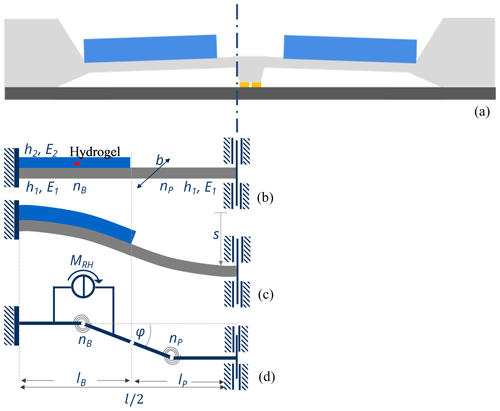Figure 2Model of the sensor switch of Fig. 1 without axial force, (a) setup, (b) bending beam partially coated with a hydrogel layer, (c) deflected sensor due to relative humidity changes, and (d) corresponding mechanical model. Shown is half of the sensor due to symmetry reasons (left: rigid clamping, right: vertically deflectable edge of the boss structure). nB, nP torsional compliance of the bending beam with and without hydrogel, respectively, l length, lB length of the hydrogel-covered part, s deflection, φ angle of deflection at the transition point between the hydrogel-coated and the uncoated part, MRH torsional moment due to the hydrogel swelling, b width, h thickness. Indices: 1 substrate (silicon, steel), 2 hydrogel, p substrate, b hydrogel/hydrogel-coated.

4 Model with axial forces

To avoid arcing and damage of the microcontact, the sensor has to switch quickly between the closed and opened state. From this, the switching characteristic needs a hysteresis. This can be achieved when the bending beam is axially compressed by means of an axial force or by applying a source of expansion. To consider switching hysteresis properties, the model of Fig. 2 is complemented by an axial force FA and an axially acting translational spring nT (Fig. 3).Figure 3Mechanical model for a pre-deflected bending beam with length l∕2, pre-deflection angle φ0, torsional spring nR, translational spring nT and humidity-dependent bending moment MRH (a) without and (b) with axial compressive force FA.

Figure 3 shows the corresponding model, based on the beam theory, of an axially compressed bending beam (Timoshenko and Gere, 2012). Here, for reasons of simplicity, the condition ${l}_{B}={l}_{P}=l/$4 is assumed. From Fig. 3a, the deflection s0 and the deflection angle φ0 of the bending beam result from a source of expansion that leads to a length expansion of the bending beam part from length lA to length lB (Fig. 3b):

$\begin{array}{}\text{(13)}& {l}_{A}=\frac{l}{\mathrm{4}}+{s}_{\mathrm{0}}\frac{l}{\mathrm{4}}\frac{\mathrm{1}}{\mathrm{cos}\left({\mathit{\phi }}_{\mathrm{0}}\right)},\text{(14)}& {l}_{B}=\frac{l}{\mathrm{4}}\frac{\mathrm{1}}{\mathrm{cos}\left(\mathit{\phi }-{\mathit{\phi }}_{\mathrm{0}}\right)},\end{array}$

where φ0 and φ are the pre-deflection angle and the change of angle.

The resulting compression Δs yields

$\begin{array}{}\text{(15)}& \mathrm{\Delta }s={l}_{B}-{l}_{A}=\frac{l}{\mathrm{4}}\left(\frac{\mathrm{1}}{\mathrm{cos}\left(\mathit{\phi }-{\mathit{\phi }}_{\mathrm{0}}\right)}-\frac{\mathrm{1}}{\mathrm{cos}\left({\mathit{\phi }}_{\mathrm{0}}\right)}\right),\end{array}$

$\begin{array}{}\text{(16)}& {F}_{A}=\frac{\mathrm{\Delta }s}{n}=\frac{\mathrm{1}}{n}\frac{l}{\mathrm{4}}\left(\frac{\mathrm{1}}{\mathrm{cos}\left(\mathit{\phi }-{\mathit{\phi }}_{\mathrm{0}}\right)}-\frac{\mathrm{1}}{\mathrm{cos}\left({\mathit{\phi }}_{\mathrm{0}}\right)}\right).\end{array}$

For the derivation of the bending moment equation at equilibrium state, FA was considered with its two vectorial components:

$\begin{array}{}\text{(17)}& {F}_{Ax}={F}_{A}\mathrm{cos}\left(\mathit{\phi }-{\mathit{\phi }}_{\mathrm{0}}\right),\text{(18)}& {F}_{Ay}={F}_{A}\mathrm{sin}\left(\mathit{\phi }-{\mathit{\phi }}_{\mathrm{0}}\right).\end{array}$

During swelling, the hydrogel-covered part acts as a bimorph providing a bending moment source MRH depending on the humidity change. From Fig. 3b, the length change of lB along the x direction results from the deflection angle φφ0. The balance of torques yields together with Eqs. (16)–(18):

$\begin{array}{}\text{(19)}& {M}_{\mathrm{RH}}-{F}_{Ay}\frac{l}{\mathrm{4}}+{F}_{Ax}\frac{l}{\mathrm{4}}\mathrm{tan}\left(\mathit{\phi }-{\mathit{\phi }}_{\mathrm{0}}\right)=\mathrm{0}.\end{array}$

For small deflection angles, the trigonometric functions in Eq. (19) can be expressed with the corresponding first terms of the Taylor series:

$\begin{array}{}\text{(20)}& \mathrm{sin}\left(x\right)=x-\frac{{x}^{\mathrm{3}}}{\mathrm{3}\mathrm{!}},\text{(21)}& \mathrm{cos}\left(x\right)=\mathrm{1}-\frac{{x}^{\mathrm{2}}}{\mathrm{2}\mathrm{!}},\text{(22)}& \mathrm{tan}\left(x\right)=x+\frac{{x}^{\mathrm{3}}}{\mathrm{3}},\end{array}$

so that Eq. (18) becomes

$\begin{array}{}\text{(23)}& {M}_{\mathrm{RH}}-{F}_{A}\frac{l}{\mathrm{4}}\left(\mathit{\phi }-{\mathit{\phi }}_{\mathrm{0}}\right)+{F}_{A}\frac{l}{\mathrm{4}}{\left(\mathit{\phi }-{\mathit{\phi }}_{\mathrm{0}}\right)}^{\mathrm{3}}=\mathrm{0}.\end{array}$

Regarding Eq. (4), MRH is proportional to εRH and, hence, to relative humidity φRH, where φRH is proportional to the deflection s. This allows us to re-write Eq. (23):

$\begin{array}{}\text{(24)}& {M}_{\mathrm{RH}}-A\left(\mathit{\phi }-{\mathit{\phi }}_{\mathrm{0}}\right)+B{\left(\mathit{\phi }-{\mathit{\phi }}_{\mathrm{0}}\right)}^{\mathrm{3}}=\mathrm{0}\end{array}$

and respectively

$\begin{array}{}\text{(25)}& {\mathit{\phi }}_{\mathrm{RH}}-{A}^{*}s+{B}^{*}{s}^{\mathrm{3}}=\mathrm{0}.\end{array}$

A and B as well as A* and B* are coefficients to be calculated from MRH and FA via Eqs. (4), (5) and (15). Figure 4a shows the curve progression of φRH versus (φφ0) for varying parameters A* and ${B}^{*}.$ For ${A}^{*}=\mathrm{1}$ and ${B}^{*}=\mathrm{0}$, the curve progression shows a linear dependency of φRH on (φφ0). For ${A}^{*}=\mathrm{1}$ and 0.2 <B*< 0.9, a hysteresis occurs and for ${A}^{*}=\mathrm{1}$ and ${B}^{*}=\mathrm{1}$ a switching without a hysteresis was found. As a consequence, by varying both the bending beam geometry and the material parameters, the shape of the curves and the width of the hysteresis can be adjusted. In Fig. 4b, the S-shaped curve progression with switching hysteresis is shown for ${A}^{*}=\mathrm{1}$ and ${B}^{*}=\mathrm{0.5}$ depending on the relative humidity with the switching points from s1 to s2 and from s3 to s4, respectively, and the curve progression without switching hysteresis for ${B}^{*}=\mathrm{1}$ and ${B}^{*}=\mathrm{0}$.Figure 4Calculated curves for (a) deflection s and (b) angle φφ0 versus relative humidity φRH. The switching points (s1s2; s3s4) are marked.

5 Fabrication of a sensor switch with switching hysteresis

To ensure that the electrical microcontacts are reliably closed and opened, oscillations between the opened and closed states as well as local electric field strength peaks that are too high and associated arcs have to be avoided. This can be achieved by implementing a switching hysteresis between two stable states (Chen et al., 2008).

To demonstrate the concept, a beam-like sensor switch was manufactured from sheet metal, an easy-to-process material, with Young's modulus of 2.06×1011 N m−2 (Fig. 5a) (DIN EN 10270-1, 2012). As shown in Fig. 5b, the bending beam was fixed in a fixation and axially clamped by means of an adjusting screw.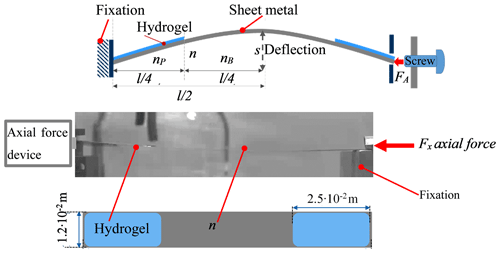Figure 5Beam-like sensor switch with switching hysteresis: (a) sensor setup with axial clamping, (b) cross-sectional and (c) top view of the setup. Hydrogel PVA/PAA, material and geometry parameters as in Table 1.

Table 1Material and geometry parameters of the beam-like sensor switch with switching hysteresis (DIN EN 10270-1, 2012; Bellmann, 2016).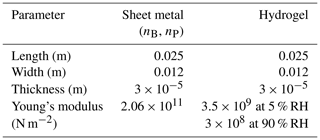The humidity-sensitive hydrogel layer (thickness $\mathrm{3}×{\mathrm{10}}^{-\mathrm{5}}$ m) was structured on the top of the metal sheet. To achieve sufficient adhesion the metal surface was pre-treated by means of a chemical cleaning process and an adhesion-promoting agent. For that, the bending beam was cleaned in a solution made of distilled H2O, 25 % ammonia and 25 % peroxide ($\mathrm{2}:\mathrm{1}:\mathrm{1}$) at 358.15 K for 900 s. Afterwards, the bending beam was pre-treated in a plasma etching system (VTD: DREVA 450) with oxygen plasma at a pressure of 15 Pa at 400 W for 300 s. Then a 3 mL solution of the adhesion-promoter agent made of 0.9 mL (3-Aminopropyl)triethoxysilane (APTES) and 99 mL H2O was applied to the plasma-treated surface and heated in an oven at 373.15 K for 600 s (Gartmann, et al., 2010).

The moisture-sensitive hydrogel was made from a blend of 15 wt % poly(vinyl alcohol) (PVA, molecular mass Mw=89 000…98 000) and 7.5 wt % poly(acrylic acid) (PAA, Mw=450 000) in a mass ratio of 4:1. Both materials were purchased from Sigma-Aldrich. The powders were solved in deionized water and mixed for several hours for PAA at 343.15 K and for PVA at 353.15 K until homogenous solutions were formed. Then both polymer solutions were mixed for several hours at 323.15 K to form the hydrogel blend (Jose et al., 2014). The hydrogel layer was deposited by a pipette of a 5 mL polymer solution, consisting of PVA/PAA, to the prepared surface with respect to the optimum coverage ratio of x=0.5 and dried at 295.15 K for 21600 s. Figure 5c shows exemplarily the structured hydrogel layer on the bending beam surface. After drying, the polymer was thermally cross-linked in an oven at 393.15 K for 1200 s (Arndt et al., 1999).

6 Deflection measurements of the sensor switch with switching hysteresis

The hydrogel-coated bending beam was axially clamped in a fixation (Fig. 5). A compression load of 0.04 N was applied by means of an adjusting screw. For its measurement, a force-measuring device (SAUTER FC 10) was applied on the opposite side of the fixation. The beam-like sensor switch was investigated in a self-made humidity chamber. The humidity was varied using a bubble system and was monitored by means of a humidity sensor (Hygro-Thermometer, B+B). The deflection of the pre-deflected bending beam was recorded via a CMOS camera (HIKVISION).

7 Experimental results

Figure 6 shows the measured deflection of the bending beam as a function of the relative humidity in the range from 5 % RH up to 95 % RH. Above 85 % RH, the bending beam abruptly switches from the pre-deflected side to the opposite side. At 53 % RH, by changing from the humid to the dry environment, the beam switches back to the initial state demonstrating successfully the switching hysteresis.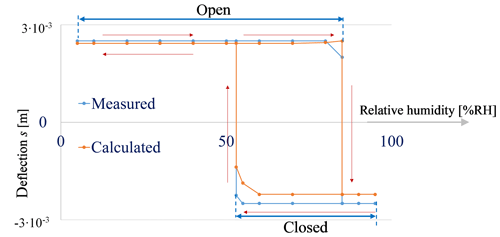Figure 6Deflection of the bending beam with structured hydrogel as function of the relative humidity. The resulting switching hysteresis shows two switching points at 53 % RH and 85 % RH.

To compare the experimental results with the mechanical model, the material properties and the geometry parameters from Table 1 are inserted into Eq. (22). The deflections of the bending beam s as function of the relative humidity are calculated from Eq. (7). In comparison to the measured data, the calculated deflection shows a deviation of 8 %, whereas the measured hysteresis width of the switching curve shows a good agreement.

8 Summary and outlook

This work deals with the development of a sensor switch with switching hysteresis for humidity sensing. The hysteresis is needed for the safe and reliable switching, i.e. fast opening and closing of the contacts. Based on the beam theory, a mechanical model was derived to calculate the deflection s depending on the degree of coverage $x={l}_{B}/l$, i.e. of the length ratio of the partly hydrogel-coated bending beam part to the total length of the bending beam. It could be shown that a maximum deflection is achieved when the stiffness of the hydrogel-coated part and the uncoated part are equal, i.e. when the lengths of the coated and uncoated parts of the beam are equal (x=0.5). To include the switching hysteresis in the mechanical model, it was complemented by an axial force FA and the axial compliance nT. From this, it could be shown that the width of the switching hysteresis is adjustable via variation of the geometry parameters, like the thickness or the length of the bending beam. To prove the mechanical model, a beam-like sensor switch was manufactured by means of a metal sheet covered with a hydrogel layer at a coverage degree of 0.5 and the switching hysteresis was successfully demonstrated.

Data availability

The data presented in this article are stored in an internal system according to the guidelines of the DFG.

Author contributions

The authors NG and GG designed and developed the experimental setup. NG and GG developed the mechanical model to describe the sensor switch. NG performed the experiments, analyzed the data, and wrote the initial paper. GG revised the paper and led the project. Both authors approved the final paper.

Competing interests

The authors declare that they have no conflict of interest.

Special issue statement

This article is part of the special issue “Sensors and Measurement Systems 2019”. It is a result of the “Sensoren und Messsysteme 2019, 20. ITG-/GMA-Fachtagung”, Nuremberg, Germany, 25–26 June 2019.

Acknowledgements

The author would like to thank his supervisor Gerald Gerlach for his support.

Financial support

The authors were supported by the Deutsche Forschungsgemeinschaft (DFG) for financial support within the Hydrogel-based Microsystems Research Training Group (GRK 1865).

Review statement

This paper was edited by Eric Starke and reviewed by two anonymous referees.

References

AMA: Sector Information: Quarter Reports 2017, available at: http://www.ama-sensorik.de/en/association/sector-information/quarterreports-2017/, last access: 14 August 2018.

Arndt, K. F., Richter, A., Ludwig, S., Zimmermann, J., Kessler, J., Kuckling, D., and Adler, H. J.: Poly (vinyl alcohol)/poly (acrylic acid) hydrogels, FT-IR spectroscopic characterization of crosslinking reaction and work at transition point, Acta Polymerica, 50, 383–390, 1999.

Bellmann, C.: Leistungslose Feuchteschwellwertschalter (Powerless Humidity Threshold Switch), Dissertation, TU Dresden, 2016.

Bellmann, C., Steinke, A., Frank, T., and Gerlach, G.: Humidity micro switch based on humidity-sensitive polymers, Proc. SPIE 9430, Electroactive Polymer Actuators and Devices (EAPAD), 94302F, 2015.

Chen, L. T., Lee, C. Y., and Cheng, W. H.: MEMS-based humidity sensor with integrated temperature compensation mechanism, Sensors and Actuat., 147, 522–528, 2008.

Dai, M., Picot, O. T., Verjans, J. M., Haan, L. T., Schenning, A. P., Peijs, T., and Bastiaansen, C. W.: Humidity-responsive bilayer actuators based on a liquid-crystalline polymer network, ACS Appl. Mater. Inter., 5, 4945–4950, 2013.

Frank, T., Steinke, A., and Gerlach, G.: Binary ZeroPower Sensors: an alternative solution for power-free energy-autonomous sensor systems, Microsyst. Technol., 18, 1225–1231, 2012.

Gartmann, N., Schütze, C., Ritter, H., and Brühwiler, D.: The effect of water on the functionalization of mesoporous silica with 3-aminopropyltriethoxysilane, J. Phys. Chem. Lett., 1, 379–382, 2010.

Huang, W. M., Lei, F., Zhao, M., and Wang, K.: A surface micromachined CMOS MEMS humidity sensor, Micromachines, 6, 1569–1576, 2015.

Jose, J., Shehzad, F., and Al-Harthi, M. A.: Preparation method and physical, mechanical, thermal characterization of poly(vinyl alcohol)/poly(acrylic acid) blends, Polym. Bull., 71, 2787–2802, 2014.

Marschner, U., Gerlach, G., Starke, E., and Lenk, A.: Equivalent circuit models of two-layer flexure beams with excitation by temperature, humidity, pressure, piezoelectric or piezomagnetic interactions, J. Sens. Sens. Syst., 3, 187–211, https://doi.org/10.5194/jsss-3-187-2014, 2014.

Rangelow, I. W., Gerlach, G., Bartuch+, H., Steinke, A., and Roeder, R.: Micromechenical sensors: switch for threshold-related switching of electrical contacts, Patentschrift: WO 2009077446 A1 25/06/2009, 2009.

Rittersma, Z. M.: Recent achievements in miniaturised humidity sensors – a review of transduction techniques, Sensors Actuat., 96, 196–210, 2002.

Norm DIN EN 10270-1: Steel wire for mechanical springs – Part 1: Patented cold drawn unalloyed spring steel wire, Beuth Verlag, 2012.

Timoshenko, S. P. and Gere, J. M.: Theory of Elastic Stability, Second edition, Newburyport, Dover Publications, 2012.